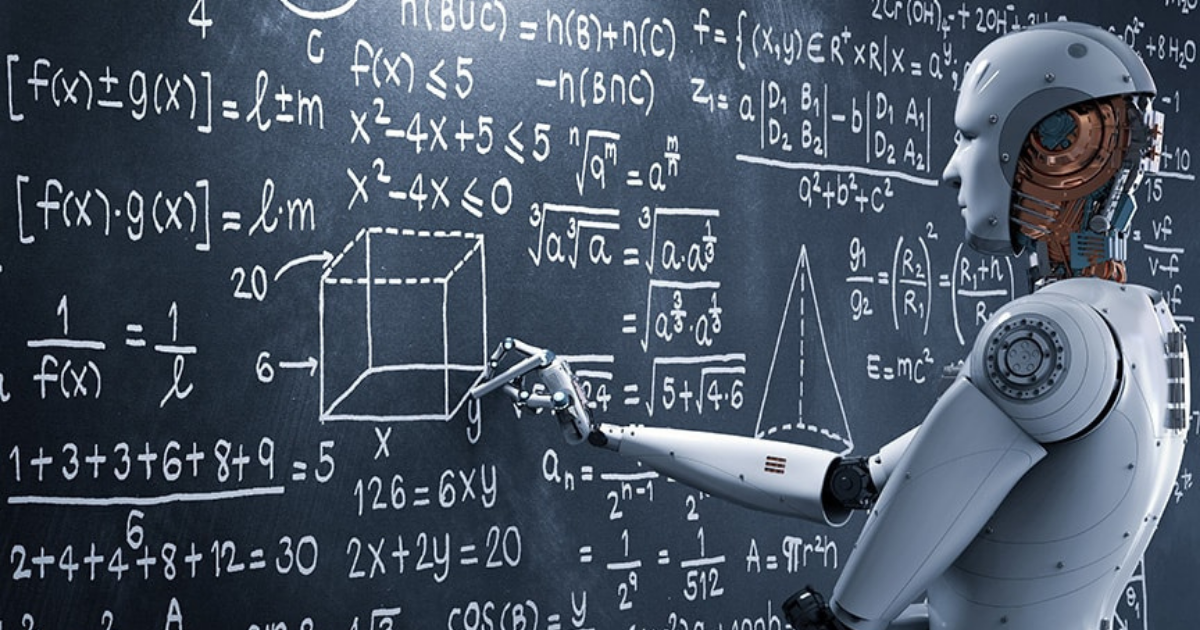Home
Blog
No items found.

# Intelligent Searching Techniques in Artificial Intelligence

Published:
May 20, 2021
Intelligent Searching Techniques in Artificial IntelligenceWe all have, at least once, lost something valuable and wondered where we left them! We frantically search our cupboards or under the bed, and every square inch of the house, even if we have not been to those parts of the house, ever. Sometimes we stop rummaging to actually think about the last place we might have kept the item.

Most probably we search again, revisit places that we didn’t search properly and skip places that we have already gone through with a fine-tooth comb. Although these methods sound like the most human thing to do, they are indeed intelligent search rules that even agents in Artificial Intelligence follow.

What are Agents in Artificial Intelligence (AI)?

The AI system is composed of agents and their environments. Agents are responsible for the actions and outcomes obtained from the system. They either use sensors to perceive the environment or actuators to act upon their environment.

How do Agents Use Various Search Techniques?

Now, when these agents are faced with a problem, they search their knowledge base for a solution. They follow multiple approaches of searching to achieve their goal, just like when we search for what we lost.

For instance, when we lose our car keys, we search every room in the house. In this instance the search space is the entire house and we are doing a random search/ blind search or what is popularly known as a brute force search in AI.

In a second scenario, assume that we have some information on where we might have left the car keys. On top of the desk in our room, on the sofa in the living room or in the cupboard. The search, then, narrows down to specific locations in the house. In an AI system this would mean that we have metadata that could help make the search quick and easy. The search space in this instance is, thus, narrower than in a blind search. This search method is known as a heuristic search.

A more improved search technique is a meta-heuristic search where the agent’s search is based on available meta information and on search experience that refines the metadata.

The search space narrows down even more after every search operation. The agents pick up on every search iteration. This approach is known as  the meta-heuristic search.

How is One Search Process Different from Another?

Different search processes find solutions to problems at different speeds. There is also a significant reduction in the search space depending on the search method followed. The average search time taken to reach a goal is directly proportional to the search space. Implying that the agents get faster search results, if, as in the case of meta-heuristic search, the search space is lesser.

More than just Speed

The popular algorithmic problem of the Travelling Salesman tries to identify the shortest and most efficient route for a salesman to take to specific destinations. While solving the travelling salesman problem using the brute force search or blind search method, the Big Ω complexity is (N-1)! where N is the number of houses a salesman travels to.

If N is a very small number (i.e. <10), it's="" still="" computable.="" but="" in="" real-life="" n="" will="" be="" somewhat="" greater="" than="" 1000.="" for="" which,="" finding="" a="" solution="" take="" lot="" of="" unnecessary="" time.="" if="" we="" solve="" it="" using="" the="" heuristic="" approach,="" then="" big="" o="" complexity="" is="" o(n2="" *="" 2n)="" with="" capabilities="" quantum="" computers,="" can="" find="" solutions="" to="" complex="" problems="" even="" through="" brute="" force="" search="" method.="" however,="" most="" real-time="" problems,="" like="" best="" possible="" container="" packing="" or="" similarity="" between="" two="" dna="" samples,="" require="" metadata="" that="" further="" expanded="" more="" iterations. ="" <="" p="">